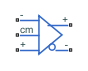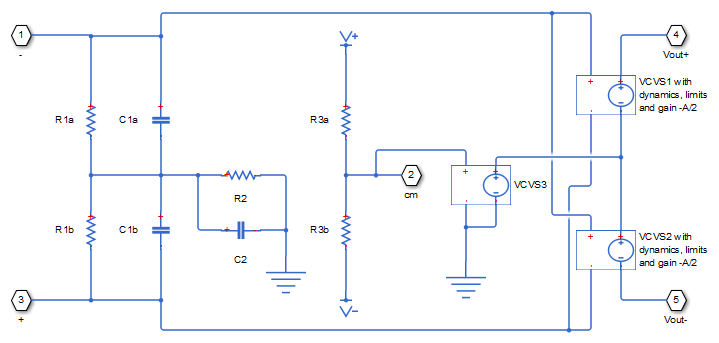# Fully Differential Op-Amp

Operational amplifier with fully differential output, that is, not referenced to ground

• Library:
• Simscape / Electrical / Integrated Circuits

•## Description

The Fully Differential Op-Amp block models a fully differential operational amplifier. Differential signal transmission is better than single-ended transmission due to reduced susceptibility to external noise sources. Applications include data acquisition where inputs are differential, for example, sigma-delta converters.

The following diagram shows the internal representation of the amplifier.Parameters for the circuit components are derived from the block parameters that you provide. The gain of the two voltage-controlled voltage sources (VCVS1 and VCVS2) is set to half of the differential gain value. Similarly the slew rate of each of the voltage sources is set to half of the differential maximum slew rate value. The voltages of the two output ports `Vout+` and `Vout-` are both limited to be within the minimum and maximum output voltages that you specify.

The output voltage for zero differential input voltage is controlled by the common-mode port, `cm`. If no current is drawn from the `cm` port by the external circuit, then the output voltage is set to be the average of the positive and negative supply voltages by the resistor ladder of R3a and R3b. Note that the negative supply voltage can be zero, which corresponds to operation when a split supply is not available. The values for the minimum and maximum output voltages that you provide must be consistent with the values for the supply voltages that you provide. So, for example, the maximum output high voltage will be less than the positive supply voltage, the difference corresponding to the number of p-n junction voltage drops in the circuit.

## Assumptions and Limitations

• This block provides a behavioral model of a fully differential operational amplifier. It does not represent nonlinear effects, such as variation in gain with output voltage amplitude, and the nonlinear nature of the output voltage-current relationship for large load currents.

.

## Ports

### Conserving

expand all

Electrical conserving port associated with the op-amp non-inverting input.

Electrical conserving port associated with the op-amp inverting input.

Electrical conserving port associated with the op-amp common-mode port. If you want to leave this pin open-circuit, connect a voltage sensor between the `cm` port and a reference port.

Electrical conserving port associated with the op-amp non-inverting output.

Electrical conserving port associated with the op-amp inverting output.

## Parameters

expand all

### Gain

The gain applied to a voltage difference between the + and – inputs.

The frequency at which the differential voltage gain drops by 3 dB from its dc value.

### Input Impedance

The input resistance seen by a voltage source applied across the + and – inputs. The default value is `1.3` MOhm.

The input capacitance seen by a current source applied across the + and – inputs.

The input resistance seen by a voltage source applied between ground and the + input, or between ground and the – input.

The input capacitance seen by a current source applied between ground and the + input, or between ground and the – input.

### Output Limits

The output resistance of either of the outputs with respect to the common-mode voltage reference. Differential output resistance is therefore twice the value of the output resistance R_out.

The minimum output voltage for either of the two output pins with respect to ground.

The maximum output voltage for either of the two output pins with respect to ground.

The maximum slew rate of the differential output voltage.

### Output Bias

The input resistance seen by a voltage source applied between ground and the common mode port.

The value of the negative supply voltage connected to common-mode bias resistor R3b (see diagram).

The value of the positive supply voltage connected to common-mode bias resistor R3a (see diagram).

### Initial Conditions

The initial differential voltage across the two outputs if the output current is zero.

## Version History

Introduced in R2012a